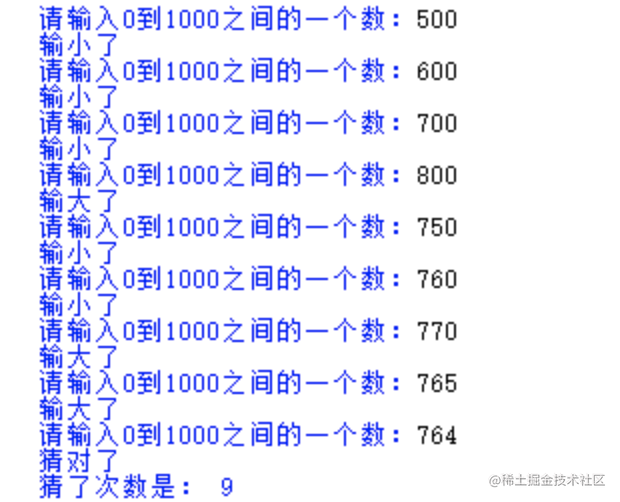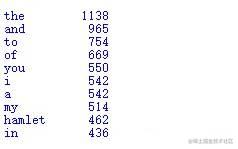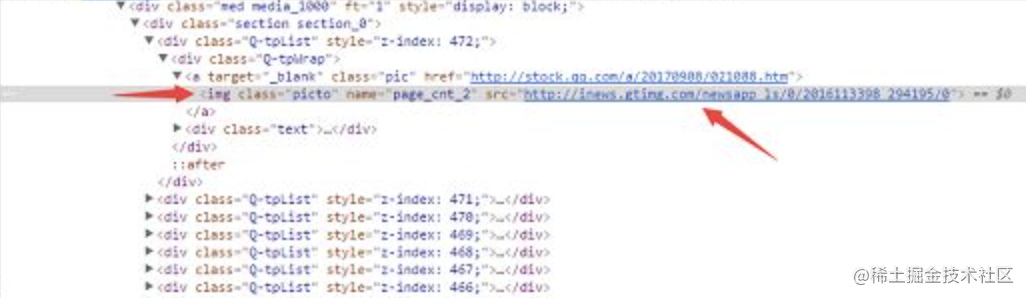为了让你们10分钟入门Python，我花5小时写了7个案例和这篇文章！！！

案例名称

• 计算圆形面积
• 输入字符并倒序输出
• 猜数字游戏
• 按照诗句格式输出诗词
• 统计文本中出现次数最多的10个单词（txt）
• web页面元素提取
• 文本进度条

计算圆形面积

print（<输出字符串模板>.format(<变量1>，<变量2>，<变量3>)）

r = 25    # 圆的半径是25
area = 3.1415 * r * r  #圆的公式
print(area)
print('{:.2f}'.format(area) )  # 只输出两位小数

format前的字符串模板格式‘{:.2f}’ 经常会写错，其中一个{}对应一个format里面的参数。

输入字符并倒序输出

• 输入使用input函数
• 计算长度使用len（）函数
• 输出函数结尾使用end=’‘，作用在输出的字符后方添加空字符串
#输入文本
s=input('请输入一段文本：')
#计算输入内容的长度并赋值给i  i=len(s)-1
#倒序循环输出
while i>=0:
print(s[i],end='')
i=i-1

猜数字游戏

• 使用random.randint()函数生成一个随机数字
• while（）循环，当未满足条件一直执行，满足条件break跳出循环
• 输入数字eval函数结合input，将字符串类型转换成整数
• if 三分支条件判断，if elif else 格式

import random
#生成随机数
a=random.randint(0,1000)
#统计次数
count=0
while True:
number=eval(input('请输入0到1000之间的一个数：'))
count=count+1
#判断比较两个数
if number>a:
print('输大了')
elif number<a:
print('输小了')
else:
print('猜对了')
break
print('猜了次数是：',count)按照诗句格式输出诗词

• 将所有标点符号替换为\n
• 文本居中对齐显示

• 替换函数line.replace(变量名，要替换的值)
• 居中对齐line.center(宽度)
• 函数调用，将文本变量txt传入替换函数linesplit中
txt = '''

'''
#定义一个函数，实现将标点符号替换为\n

def linesplit(line):
plist = [',', '!', '?', '，', '。', '！', '？']
for p in plist:
line=line.replace(p,'\n')

return line.split('\n')
linewidth = 30  # 预定的输出宽度

#定义一个函数，实现居中对齐
def lineprint(line):
global linewidth
print(line.center(linewidth))
#调用函数

newlines=linesplit(txt)
for newline in newlines:
lineprint(newline)

统计文本中出现次数最多的10个单词• 首先，将文本内容统一为小写，使用lower()函数
• 再次，将文本中特殊字符替换为空格，replace()函数
• 按空格将文本进行切割，使用split（）函数
• 统计单词出现的次数
• 按频率从大到小排序 sort()函数
• 按照固定格式输出 ，使用format()函数

def gettxt():
#读取文件
txt=txt.lower()

for ch in ''!"#\$%&()*+,-./:;<=>?@[\]^_‘{|}~':'
txt=txt.replace('')
return txt

hmlttxt=gettxt()
words=hmlttxt.split()

counts=0
for word in words:
counts[word]=counts.get(word,0)+1
#对word出现的频率进行统计，当word不在words时，返回值是0，当word在words中时，返回+1，以此进行累计计数

items=list(counts.items())

for i in range(10)
word,count=item[i]
print('{0:<10}{1:>5}'.format(word,count))

# 首先，将文本内容统一为小写，使用lower()函数
def gettxt():
txt=txt.lower()
# 将文本中特殊字符替换为空格，replace()函数
for ch in '!"#\$%&()*+,-./:;<=>?@[\]^_‘{|}~':
txt=txt.replace(ch,'')
return txt
# 按空格将文本进行切割，使用split（）函数
hamlettxt=gettxt()
words=hamlettxt.split()
# 统计字数

counts={}
for word in words:
counts[word]=counts.get(word,0)+1

# 按频率从大到小排序  sort()函数
items=list(counts.items())
items.sort(key=lambda x:x,reverse=True)
# 按照固定格式输出 ，使用format()函数

for i in range(10):
word, count=items[i]
print("{0:<10},{1:>5}".format(word,count))

web页面元素提取图片url路径信息

• 首先，提取页面所有元素
• 其次，提取图片的url路径
• 然后，将路径信息输出显示
• 最后，将这些路径保存到文件中

• 提取页面所有元素

def gethtmllines(htmlpath):
#文件打开
f=open(r,'htmlpath',encoding='utf-8')
#文件关闭
f.close()
return ls

• 提取图片的url路径• 列表形式存放截取后的地址
• 列表的切割，split()函数
def geturl(ls):
urls=[]
for line in ls:
if 'img' in line:
url=line.split('src=')[-1].split('"')
urls.append(url)

• 将路径信息输出显示

for循环将路径信息输出
def show(urls):
count=0
for url in urls:
print('第{:2}个url{}'.format(count,url))
count+=1

• 将这些路径保存到文件中

def save(filepath,urls):
f=open(filepate,'w')
for url in urls:
f.write(url+'\n')
f.close()

• main()函数，将上面的函数进行组合
def main():
inputfile  = 'nationalgeographic.html'
outputfile = 'nationalgeographic-urls.txt'
htmlLines = getHTMLlines(inputfile)
imageUrls = extractImageUrls(htmlLines)
showResults(imageUrls)
saveResults(outputfile, imageUrls)

# Example_8_1.py
#1. 按行读取页面所有内容
def getHTMLlines(htmlpath):
f = open(htmlpath, "r", encoding='utf-8')
f.close()
return ls

#2. 提取http路径
def extractImageUrls(htmllist):
urls = []
for line in htmllist:
if 'img' in line:
url = line.split('src=')[-1].split('"')
print
if 'http' in url:
urls.append(url)
return urls

#3. 输出链接地址
def showResults(urls):
count = 0
for url in urls:
print('第{:2}个URL:{}'.format(count, url))
count += 1

#4. 保存结果到文件
def saveResults(filepath, urls):
f = open(filepath, "w")
for url in urls:
f.write(url+"\n")
f.close()

def main():
inputfile  = 'nationalgeographic.html'
outputfile = 'nationalgeographic-urls.txt'
htmlLines = getHTMLlines(inputfile)
imageUrls = extractImageUrls(htmlLines)
showResults(imageUrls)
saveResults(outputfile, imageUrls)
main()

文本进度条

• 引入time库
• print()输出格式
• 其中运用到了for i in range():循环，在循环末尾通过format函数，将其内的值赋予槽中。

import time

def bar(scale):
print('===========执行开始============')
for i in range(scale + 1):
a = '*' * i
b = '.' * (scale - i)
c = (i / scale) * 100
print('\r{:^3.0f}%[{}->{}]'.format(c, a, b), end = '')
time.sleep(0.1)
print('\n===========执行结束============')

总结

• input函数实现输入
• print结合format（）函数对结果进行输出
• 计算字符串长度len()函数
• 使用random.randint()函数生成随机数字
• eval函数结合input，将字符串类型转换成整数
• if 三分支条件判断，if elif else 格式
• 替换函数line.replace(变量名，要替换的值)
• 文本内容统一为小写，使用lower()函数
• 文本中特殊字符替换为空格，replace()函数
• 文本进行切割，使用split（）函数
• 从大到小排序 sort()函数

软件测试人员为什么选择学习Python不是Java

Python 语法简洁而清晰，并具有丰富和强大的类库，可以轻易实现很多功能。对于初学编程者来说，Python 是最好的入门语言，没有之一。

• UI自动化测试（Python+Selenium等）
• 接口测试(Python requests等)
• 性能测试(Python Locust等)
• 安全性测试(Python Scapy等)
• 兼容性测试(Python+Selenium等)

收藏时间

python自动化测试专属视频、Python自动化详细资料、全套面试题等知识内容。大家有需要可以请点击此处入群自取，希望能帮到你。

• ✔️我做了一个公众号 程序员一凡 ，不定期分享学习干货
• ✔️如果这篇文章对你有用，记得点个赞👍🏻加个关注，你的每一个点赞我都认真的当成了喜欢~
• ✔️我们下期见！👋👋👋